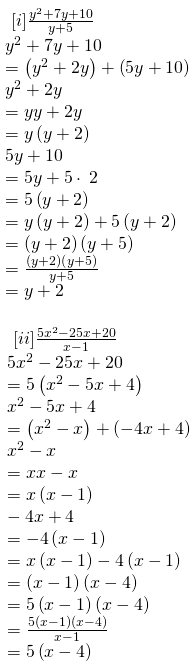# RBSE Maths Class 8 Chapter 10: Important Questions and Solutions

RBSE Maths Chapter 10 – Factorization Class 8 Important questions and solutions can be used as a reference to face the board examination. All the important questions, additional questions and solutions covering all the concepts of Chapter 10, available at BYJU’S, contain step by step answers. All these important questions are based on the new pattern designed by the RBSE. Students can also get the syllabus and textbooks on RBSE Class 8 solutions.

Chapter 10 of the RBSE Class 8 Maths will help the students to solve problems related to factors, factors of algebraic expressions, method of factorization, method of common factors, factorization by regrouping terms, factorization using identities, division of algebraic expressions.

### RBSE Maths Chapter 10: Exercise 10.1 Textbook Important Questions and Solutions

Question 1: Find the common factors of the given terms.

[i] 12x, 36

[ii] 14pq, 28p2q2

[iii] 6abc, 24ab2, 12a2b

[iv] 16x3, -4x2, 32x

[v] 10pq, 20qr, 30rp

[vi] 3x2y3, 10x2y2, 6x2y2z

Solution:

[i] 12x, 36

12x = 2 * 2 * 3 * x

36 = 2 * 2 * 3 * 3

The common factors of 12x and 36 are 2 * 2 * 3 = 12

[ii] 14pq, 28p2q2

14pq = 2 * 7 * p * q

28p2q2 = 2 * 7 * 2 * p * p * q * q

The common factors of 14pq, 28p2q2 are 2 * 7 * p * q = 14pq

[iii] 6abc, 24ab2, 12a2b

6abc = 2 * 3 * a * b * c

24ab2 = 2 * 2 * 2 * 3 * a * b * b

12a2b = 2 * 2 * 3 * a * a * b

The common factors of 6abc, 24ab2, 12a2b are 2 * 3 * a * b = 6ab

[iv] 16x3, -4x2, 32x

16x3 = 2 * 2 * 2 * 2 * x * x * x

-4x2 = (-1) * 2 * 2 * x * x

32x = 2 * 2 * 2 * 2 * 2 * x

The common factors of 16x3, -4x2, 32x are 2 * 2 * x = 4x

[v] 10pq, 20qr, 30rp

10pq = 2 * 5 * p * q

20qr = 2 * 5 * 2 * q * r

30rp = 2 * 3 * 5 * r * p

The common factors of 10pq, 20qr, 30rp are 2 * 5 = 10

[vi] 3x2y3, 10x2y2, 6x2y2z

3x2y3 = 3 * x * x * y * y * y

10x2y2 = 2 * 5 * x * x * y * y

6x2y2z = 2 * 3 * x * x * y * y * z

The common factors of 3x2y3, 10x2y2, 6x2y2z are x * x * y * y = x2y2

Question 2: Factorise the following expressions by common factors method.

[i] 6p – 12q

[ii] 7a2 + 14a

[iii] 10a2 – 15b2 + 20c2

[iv] ax2y + bxy2 + cxyz

[v] x2yz + xy2z + xyz2

[vi] -16z + 20z3

Solution:

[i] 6p – 12q

6 is taken commonly from the terms,

= 6 (p – 2q)

[ii] 7a2 + 14a

7a is taken commonly from the terms,

= 7a (a + 2)

[iii] 10a2 – 15b2 + 20c2

5 is taken commonly from the terms,

= 5 (2a2 – 3b2 + 4c2)

[iv] ax2y + bxy2 + cxyz

xy is taken commonly from the terms,

= xy (ax + by + cz)

[v] x2yz + xy2z + xyz2

xyz is taken commonly from the terms,

= xyz (x + y + z)

[vi] -16z + 20z3

= (4z) (-4 + 5z2)

Question 3: Factorise the following expressions by regrouping method.

[i] 2xy + 3 + 2y + 3x

[ii] z – 7 – 7xy + xyz

[iii] 6xy – 4y + 6 – 9x

[iv] 15pq + 15 + 9q + 25p

Solution:

[i] 2xy + 3 + 2y + 3x

= 2xy + 2y + 3 + 3x

= 2y (x + 1) + 3 ( 1 + x)

= (2y + 3) (x + 1)

[ii] z – 7 – 7xy + xyz

= z – 7 – xy (7 – z)

= 1 (z – 7) + xy ( z – 7)

= (1 + xy) (z – 7)

[iii] 6xy – 4y + 6 – 9x

= 2y (3x – 2) – 3 (-2 + 3x)

= (2y – 3) (3x – 2)

[iv] 15pq + 15 + 9q + 25p

= 15pq + 9q + 15 + 25p

= 3q (5p + 3) + 5 (3 + 5p)

= (3q + 5) (3 + 5p)

### RBSE Maths Chapter 10: Exercise 10.2 Textbook Important Questions and Solutions

Question 1: Factorize the following expressions.

[i] a2 – 4

[ii] a2 – 49b2

[iii] p3 – 121p

[iv] (a – b)2 – c2

[v] a4 – b4

[vi] 5x3 – 125x

[vii] 63a2 – 112b2

[viii] 9x2y2 – 16

[ix] (l + m)2 – (l – m)2

Solution: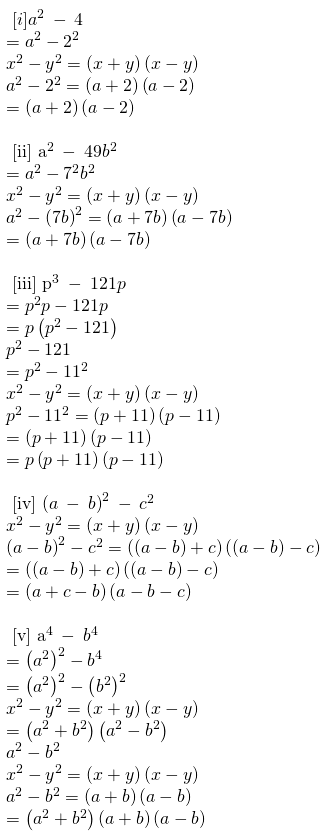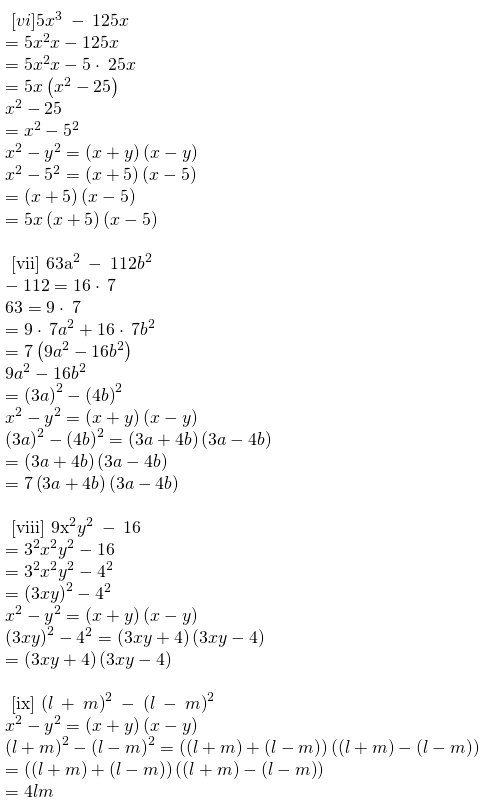Question 2: Factorise the following expressions.

[i] lx2 + mx

[ii] 2x3 + 2xy2 + 2xz2

[iii] a (a + b) + 4 (a + b)

[iv] (xy + y) + x + 1

[v] 5a2 – 15a – 6c + 2ac

[vi] am2 + bm2 + bn2 + an2

Solution: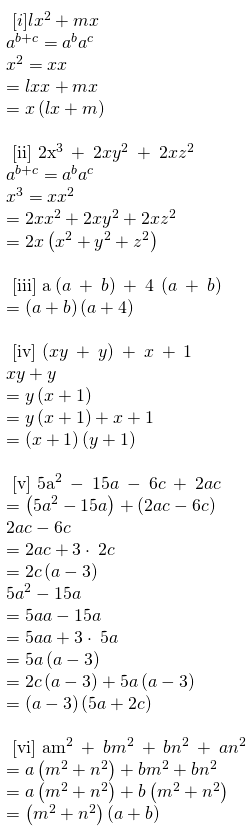Question 3: Factorise the following expressions.

[i] x2 + 5x + 6

[ii] q2 + 11q + 24

[iii] m2 – 10m + 21

[iv] x2 + 6x – 16

[v] x2 – 7x – 18

[vi] k2 – 11k – 102

[vii] y2 + 2y – 48

[viii] d2 – 4d – 45

[ix] m2 + 16m + 63

[x] n2 – 19n – 92

[xi] p2 – 10p + 16

[xii] x2 + 4x – 45

Solution: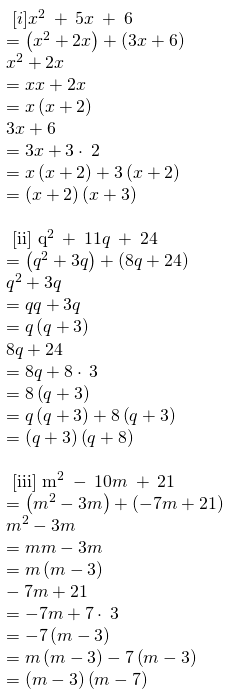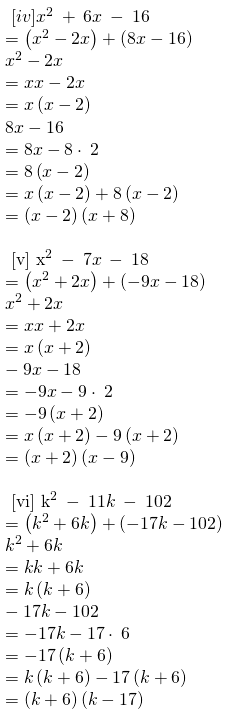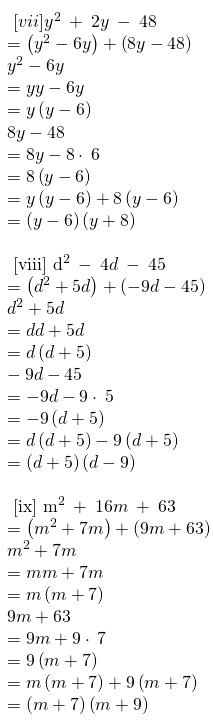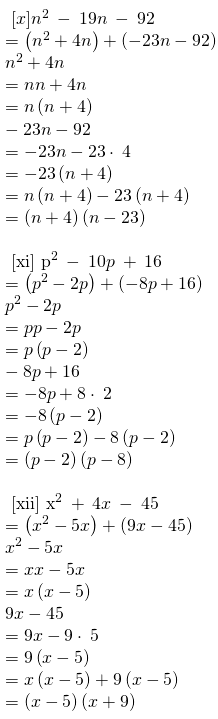### RBSE Maths Chapter 10: Exercise 10.3 Textbook Important Questions and Solutions

Question 1: Carry out the following divisions.

[i] 28x4 ÷ 56x

[ii] -36y3 ÷ 9y2

Solution: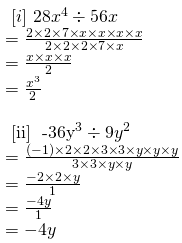Question 2: Divide the given polynomial by the given monomial

[i] (5x2 – 6x) by 3x

[ii] (x3 + 2x2 + 3x) by 2x

Solution: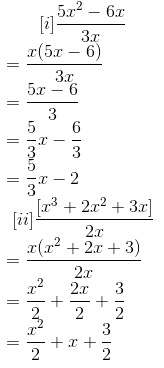Question 3: Solve the following.

[i] 10y (6y + 21) ÷ 5(2y + 7)

[ii] 9x2y2 (3z – 24) ÷ 27xy (z – 8)

Solution: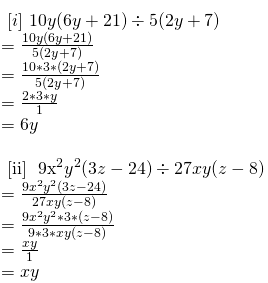Question 4: Factorise the given expressions and divide them as directed.

[i] (y2 + 7y + 10) ÷ (y + 5)

[ii] (5x2 – 25x + 20) ÷ ( x – 1)

Solution: